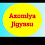## Saturday, October 20, 2007

### Multiple Choice Questions on Thermoelectric Effect

Questions involving neutral temperature and temperature of inversion often appear in entrance examination question papers. You have to remember that the neutral temperature is a constant for a given thermo couple where as the temperature of inversion is not a constant. The temperature of inversion is dependent on the temperature of the cold junction and is always as much above the neutral temperature as the cold junction is below it.

Now, consider the following MCQ:

The temperature of the cold junction of a thermocouple is 0º C and its neutral temperature is 275º C. If the temperature of the cold is changed to 20º C, the neutral temperature and the temperature of inversion will be respectively

(a) 265º C and 550º C (b) 265º C and 530º C (c) 275º C and 530º C

(d) 275º C and 550º C (e) 275º C and 510º C

The neutral temperature will be unchanged (275º C). Since the cold junction is 255º C below the neutral temperature, the temperature of inversion has to be 255º C above the neutral temperature and will be 530º C. So, the correct option is (c).

Consider now the following simple question:

The metal which does not exhibit Thomson effect is

(a) iron (b) nickel (c) copper

The correct option is (d). In determining thermo electric quantities, lead is often used as one member of the couple because of the absence of Thomson effect in it.

What is the unit of thermo electric power?

The term ‘thermoelectric power’ is a misnomer. Thermoelectric power is dV/dT where V is the thermo emf and T is the temperature of the hot junction. There is no ‘power’ involved in it and the unit is volt per Kelvin.

1.Dear Mr. MV

You are doing a very useful job. I just came to know of the blog and visited.

As a parent, I wanted to help my child and started relearning physics, chemistry and mathematics along with my current subject of teaching investments and finance.

I am documenting my efforts in blogs like www.iit-jee-chemistry.blogspot.com

I also started an orkut community for students, teachers and parents
http://www.orkut.com/Community.aspx?cmm=39291603

I shall post in my blog as well various orkut community regarding your blog.

Thank you
Narayana Rao

2.Dear Mr Narayana Rao,
Thank you very much for your words of appreciation. I have been very busy during the past couple of weeks. I will definitely visit your blogs related to Physics whenever time permits.
MV

3.the EMF of a Thermocouple, one junction of which is kept at zero degree celsius is given by E= bt+ Ct2. Find the neutral temperature and the peltier and Thomson coefficient. Give me the answer of this question

4.Here is the answer for Tech solve:
The expression for thermo emf ‘E’ in the present case is
E = b(T - 273) + c(T - 273)2 where T is the temperature of hot junction in Kelvin.
At neutral temperature dE/dT = 0
Therefore, b + 2c(Tn - 273) = 0 where Tn is the neutral temperature in Kelvin.
This gives (Tn - 273) = - b/2c
The neutral temperature tn in degree Celsius = - b/2c
Peltier coefficient π = T dE/dT = T[b + 2c(T - 273)] = T(b + 2ct) where t is the temperature of hot junction in degree Celsius.
Thomson coefficient σ = T (d2E/dT2) = 2cT